# Ncert Class 6 Math Basic Geometrical Ideas Exercise 4.1

Basic Geometrical Ideas Class 6 Ex. 4.1

Ncert Class 6 Math Free Solution.

Exercise 4.1

Question 1 :-Use the figure to name: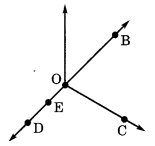(a) Five points
(b) A line
(c) Four rays
(d) Five line segments

Solution 1:-

(a) Five points are :- O, B, C, E and D.

Some are :- DE or DO or DB or EO etc.

Some are :- DB or DE or OB or OB or EB or OE etc.
Four rays are: OC, OB, OE, and OD.

Some are :- DE or DO or EB or OB or EO etc.
Five line segments are:
OE, ED, OD, OB and EB.

Question 2 :- Name the line given in all possible (twelve) ways, choosing only two letters at a times from the four given.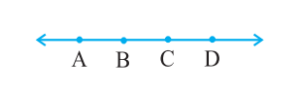Solution 2:-
The following names can be given to the lines: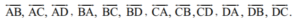Question 3 :- Use the figure to name: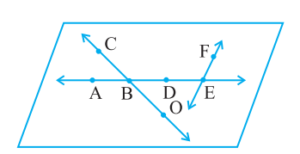(a) Line containing point E.
(b) Line passing through A.
(c) Line on which 0 lies.
(d) Two pairs of intersecting lines.

Solution 3:-
(c) CO or OC.
(d) Many answer are possible. Some are CO, AE or AE, EF.

Question 4 :- How many lines can pass through
(a) one given point?
(b) two given points?

Solution 4 :-
(a) Infinite number of lines can pass through to the given one point.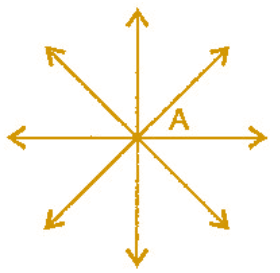(b) Only one line can pass through to the given two points.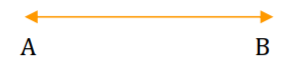Question 5 :- Draw a rough figure and label suitably in each of the following cases:
(a) Point P lies on AB.
(b) XY and PQ intersect at M.
(c) The line L contains E and F but not D.
(d) OP and OQ meet at O.

Solution 5:-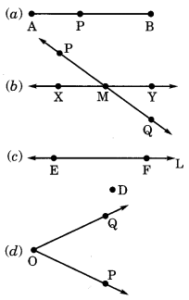Question 6 :- Consider following figure of the line MN. Say whether following statements are true or false in context of the given figure.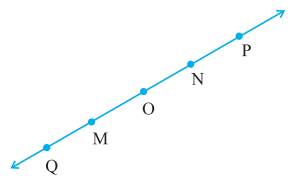(a) Q, M, O, N, P are the points on the line MN.
(b) M, O, N are the points on a line segment MN.
(c) M and N are the end points of line segment MN.
(d) O and N are the end points of line segment OP.
(e) M is one of the end points of the line segment QO.
(f) M is point on ray OP.
(g) Ray OP is different from ray QP.
(h) Ray OP is the same as ray OM.
(i) Ray OM is not opposite to the ray OP.
(j) O is not an initial point of OP.
(k) N is  initial point of NP and NM.
Solution 6:-

(a) True
(b) True
(c) True
(d) False
(e) False
(f) False
(g) True
(h) False
(i) False
(j) False
(k) True